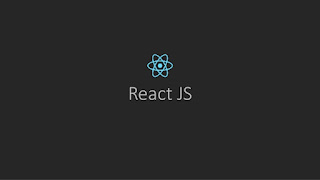### Late static binding in PHP

As of PHP 5.3.0, PHP implements a feature called late static bindings which can be used to reference the called class in a context of static inheritance.

self keyword you use to access static properties and methods Self is resolved at compile time, which means that when class is inherited from another class, self will always relate to the class where it is mentioned not the class which is inheriting it.

so lets look an example of self :

```class A {

public static function getClass()
{
echo __CLASS__;
}

public static function callClass()
{
//reference of A::getClass here
self::getClass();
}

}

class B extends A{

public static function getClass() {
echo __CLASS__;
}

}

//so what will be the output of this code
B::callClass(); //A
```

As we seen self is reffering to the class A (Base Class) in above example. B::callClass() will call the A::getClass because (self will always relate to the class where it is mentioned A::getClass() not the class which is inheriting it) so to solve this problem we use late static binding  . The late static binding will overcome this problem by using static keyword that  reference the class that was initially called at runtime. so call it statically instead of using self here .

```class A {

public static function getClass()
{
echo __CLASS__;
}

public static function callClass()
{
//reference of A::getClass here
static::getClass();
}
}

class B extends A{
public static function getClass() {
echo __CLASS__;
}

}

//so what will be the output of this code
B::callClass(); //B
```